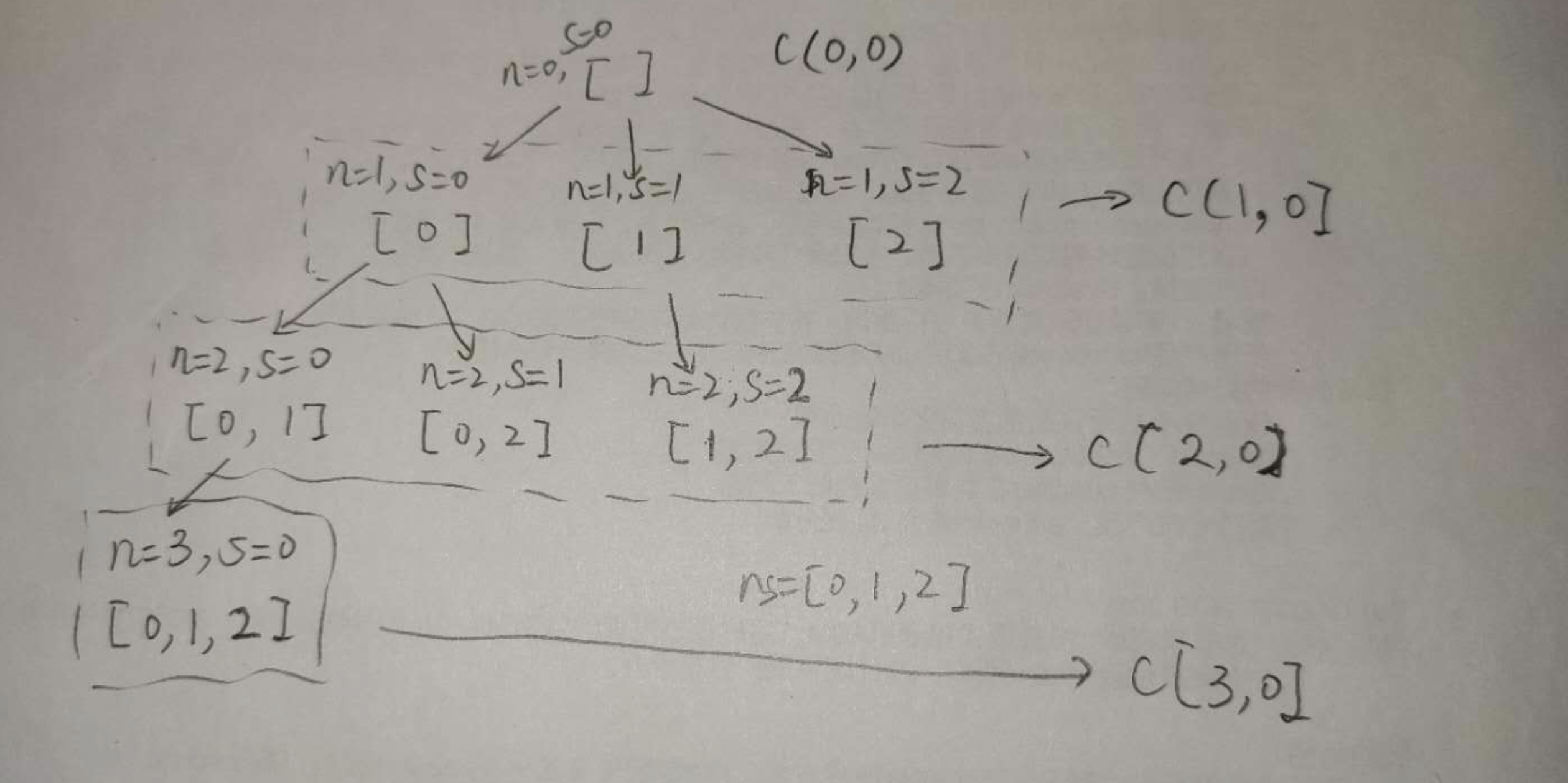# ARTS 打卡 Week 03teokingAlgorithm: 每周至少做一个 LeetCode 的算法题

Review: 阅读并点评至少一篇英文技术文章

Tips: 学习至少一个技术技巧

Share: 分享一篇有观点和思考的技术文章

﻿

# Algorithm

LC 78 Subsets

class Solution {    int[] nums;    List<List<Integer>> answer;    List<List<Integer>> subsets(int[] nums) {        answer = new ArrayList<>();        this.nums = nums;        Stack<Integer> cur = new Stack<>();        // 求c(0, 0), c(1, 0), ..., c(n, 0)，其和就是最终结果        for (int i = 0; i <= nums.length; ++i) {            dfs(i, 0, cur);        }        return answer;    }    /**     * 求从start开始，取n个元素的组合数     * @param n 需要取多少个元素     * @param start 当前取值的起始位置     * @param cur 当前取值数组     */    private void dfs(int n, int start, Stack<Integer> cur) {        // 当前取值数组大小等于需要取元素的个数        if (cur.size() == n) {            answer.add(new ArrayList<>(cur));            return;        }        for (int i = start; i < nums.length; ++i) {            cur.push(nums[i]);            dfs(n, i + 1, cur);            cur.pop();        }    }}combination运行流程

﻿

﻿

# Tips

iOS的xib编辑器，在某些情况下，打开后特别卡（开发机为内存16gb，ssd 2019款的mbp），调试效率很低。目前不知道其原因。

﻿

# Share

﻿

﻿### teoking

Monkey plays software. 2018.11.28 加入

## 评论